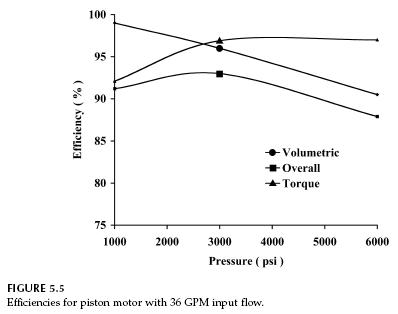﻿ Hydraulic Piston Motor Efficiency – Hydraulic Schematic Troubleshooting

## Hydraulic Piston Motor Efficiency

The fixed displacement axial piston motor (Sauer-Danfoss Model 90-075 MF), comparable to the variable displacement axial piston pump (Sauer-Danfoss Model 90-075 PV), was chosen. This motor has a maximum speed of 3950 rpm and a rated pressure of 6000 psi. Displacement is 4.57 in3/rev. Efficiencies were calculated for a 36 GPM input flow.

Torque efficiency for this design (Fig. 5.5) increases to a maximum at 3000 psi and remains constant at higher pressures. Volumetric efficiency decreases from 99% at 1000 psi to 90.5% at 6000 psi. For comparison, the volumetric efficiency of the equivalent pump decreases from 99% at 1000 psi to 90.5% at 6000 psi. At 2500 psi, the overall efficiency of the motor is 92.5% and for the pump it is 93.3%. If this pump and motor are used as a hydrostatic transmission, the overall efficiency (mechanical energy out divided by mechanical energy in), neglecting line losses, is

0.933 × 0.925 = 0.86